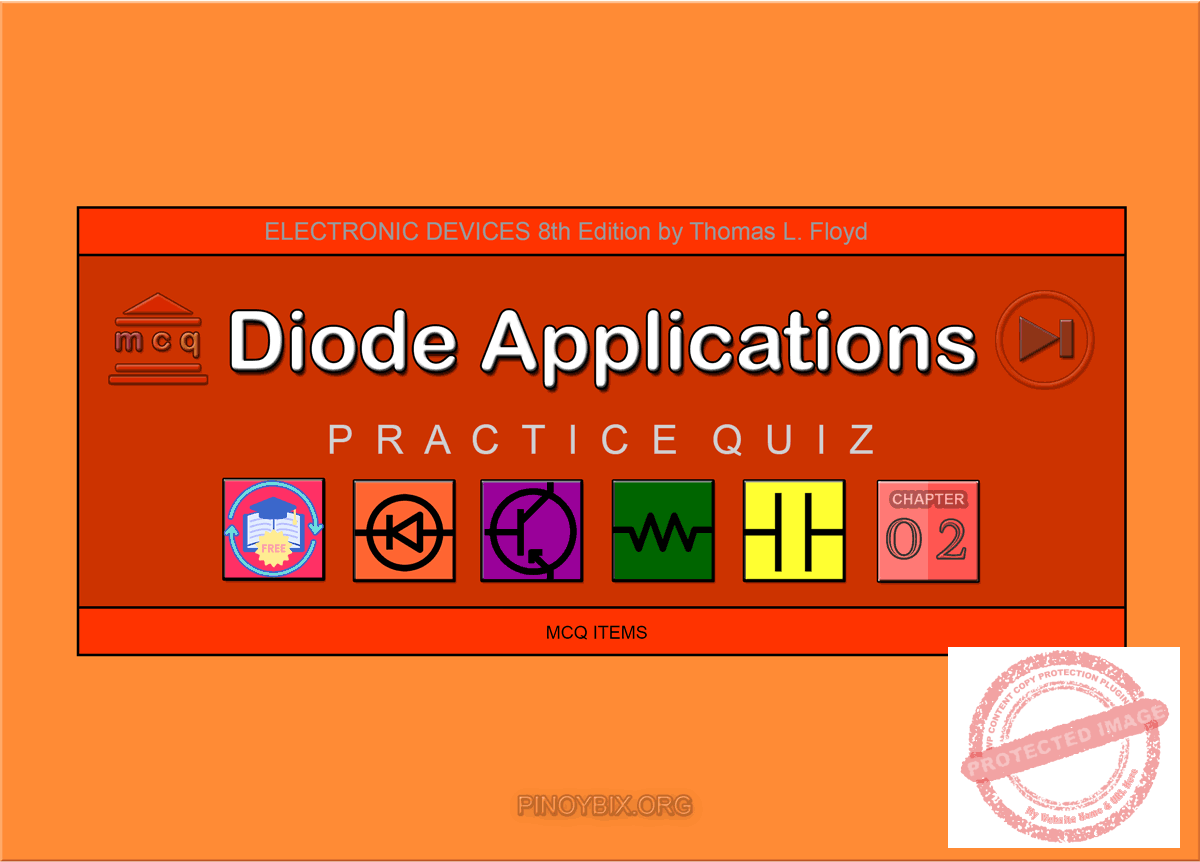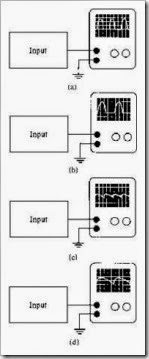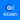# Floyd: MCQ in Diode Applications

(Last Updated On: December 5, 2019)This is the Multiple Choice Questions in Diode Applications from the book Electronic Devices – Electron Flow Version and Conventional Current Version 8th Edition by Thomas L. Floyd. If you are looking for a reviewer in Electronics Engineering this will definitely help. I can assure you that this will be a great help in reviewing the book in preparation for your Board Exam. Make sure to familiarize each and every questions to increase the chance of passing the ECE Board Exam.

#### Online Questions and Answers Topic Outline

• MCQ in Diode Operation
• MCQ in Voltage-Current (V-I) Characteristics of a Diode
• MCQ in Diode Models
• MCQ in Half-Wave Rectifiers
• MCQ in Full-Wave Rectifiers
• MCQ in Power Supply Filters and Regulators
• MCQ in Diode Limiters and Clampers
• MCQ in Voltage Multipliers
• MCQ in The Diode Datasheet
• MCQ in GreenTech Application 2: Solar Power

If you are looking for the Self-test in Floyd’s Electronic Devices proceed to

#### Practice Exam Test Questions

Choose the letter of the best answer in each questions.

1. If the ac supply is 60 Hz, what will be the ripple frequency out of the half-wave rectifier?

A) 30 Hz

B) 50 Hz

C) 60 Hz

D) 120 Hz

Solution:

2. If the ac supply is 50 Hz, what will be the ripple frequency out of the full-wave rectifier?

A) 50 Hz

B) 60 Hz

C) 100 Hz

D) 120 Hz

Solution:

3. A silicon diode in a half-wave rectifier has a barrier potential of 0.7 V. This has the effect of

A) reducing the peak output voltage by 0.7 V.

B) increasing the peak output voltage by 0.7 V.

C) reducing the peak input voltage by 0.7 V.

D) no effect.

Solution:

4. In a regulated supply, what term describes how much change occurs in the output voltage for a given change in the input voltage?

B) voltage regulator

C) line regulation

D) ripple voltage

Solution:

5. In a regulated supply, what term describes how much change occurs in the output voltage over a certain range of load current values, from minimum to maximum current?

A) line regulation

B) voltage regulator

C) current regulator

Solution:

6. PIV is which of the following?

A) peak input voltage

B) peak inverse voltage

C) peak immediate voltage

D) positive input voltage

Solution:

7. Refer to Figure 2-1. The probable trouble, if any, indicated by these voltages is

Figure 2-1

A) one of the diodes is open.

B) a diode is shorted.

C) an open transformer secondary.

D) the filter capacitor is shorted.

E) no trouble exists.

Solution:

8. Refer to Figure 2-1. If the voltmeter across the transformer reads 0 V, the probable trouble, if any, would be

A) one of the diodes is open.

B) a diode is shorted.

C) an open transformer secondary.

D) the filter capacitor is shorted.

E) no trouble exists.

Solution:

9. Refer to Figure 2-2. Which oscilloscope trace indicates the output from a properly operating half-wave rectifier without a filter?Figure 2-2.
Assume that each scope has the same settings
Assume that each scope has the same settings

A) a

B) b

C) c

D) d

Solution:

10. Refer to Figure 2-2. Which oscilloscope trace indicates the output from a properly operating full-wave rectifier with a filter?

A) a

B) b

C) c

D) d

Solution:

11. Refer to Figure 2-2. Which oscilloscope trace indicates the output from a full-wave filtered rectifier with an open diode?

A) a

B) b

C) c

D) d

Solution:

12. Refer to Figure 2-2. The oscilloscope trace in (b) could represent the output from

A) a full-wave rectifier (no filter) with an open diode.

B) a normal half-wave power supply.

C) a filtered full-wave rectifier with an open diode.

D) a full-wave rectifier with a shorted diode.

Solution:

13. Refer to the circuit in Figure 2-3(a). Refer to the output waveforms shown in Figure 2-4 and select the correct approximate output waveform.

B) b

C) c

D) d

Solution:

14. Refer to the circuit in Figure 2-3(b). Refer to the output waveforms shown in Figure 2-4 and select the correct approximate output waveform.

A) a

B) b

C) c

D) d

Solution:

15. Refer to the circuit in Figure 2-3(c). Refer to the output waveforms shown in Figure 2-4 and select the correct approximate output waveform.

A) a

B) b

C) c

D) d

Solution:

16. Refer to the circuit in Figure 2-3(d). Refer to the output waveforms shown in Figure 2-4 and select the correct approximate output waveform.

A) a

B) b

C) c

D) d

Solution:

17. Refer to Figure 2-5. Which diode arrangement will supply a negative output voltage?

A) a

B) b

C) c

D) d

Solution:

18. Refer to Figure 2-5. Which diode arrangement will supply a positive output voltage?

A) a

B) b

C) c

D) d

Solution:

19. A silicon diode has a voltage to ground of –117 V from the anode. The voltage to ground from the cathode is –117.7 V. The diode is

A) open.

B) shorted.

C) forward-biased.

D) reverse-biased.

Solution:

20. The output frequency of a half-wave rectifier is _____ the input frequency.

A) one-half

B) twice

C) equal to

D) none of the above

Solution:

21. Each diode in a center-tapped full-wave rectifier is _____ -biased and conducts for _____ of the input cycle.

A) forward, 90°

B) reverse, 180°

C) forward, 180°

D) reverse, 90°

Solution:

22. The output frequency of a full-wave rectifier is _____ the input frequency.

A) one-half

B) equal to

C) twice

D) one-quarter

Solution:

23. What is the PIV for each diode in a full-wave center-tapped rectifier? Note: Vp(out) = peak output voltage.

A) Vp(out) – 0.7 V

B) Vp(out) + 0.7 V

C) 2Vp(out) – 0.7 V

D) 2Vp(out) + 0.7 V

Solution:

24. In the operation of a half-wave rectifier with a capacitor-input filter, the ripple factor can be lowered by _____ the value of the filter capacitor or _____ the load resistors.

A) decreasing, decreasing

B) decreasing, increasing

C) increasing, decreasing

D) increasing, increasing

Solution:

25. What type of diode circuit is used to clip off portions of signal voltages above or below certain levels?

A) clipper or limiter

B) clamper

C) IC voltage regulator

D) none of the above

Solution:

26. What type of diode circuit is used to add or restore a dc level to an electrical signal?

A) clipper or limiter

B) clamper

C) IC voltage regulator

D) none of the above

Solution:

27. What is the VRRM (PIV rating) for the 1N4001 rectifier diode?

A) 50 V

B) 100 V

C) 200 V

D) 400 V

E) none of the above

Solution:

28. How many terminals do the 7800 series fixed positive voltage regulators have?

A) 2

B) 3

C) 4

D) 5

E) none of the above

Solution:

### Complete List of Chapter Quiz in Floyd’s Electronic Devices

Help Me Makes a Difference!

 P inoyBIX educates thousands of reviewers/students a day in preparation for their board examinations. Also provides professionals with materials for their lectures and practice exams. Help me go forward with the same spirit. “Will you make a small gift today via GCASH?”+63 966 459 6474 Option 1 : \$1 USD Option 2 : \$3 USD Option 3 : \$5 USD Option 4 : \$10 USD Option 5 : \$25 USD Option 6 : \$50 USD Option 7 : \$100 USD Option 8 : Other Amount© 2014 PinoyBIX Engineering. © 2019 All Rights Reserved | How to Donate? |